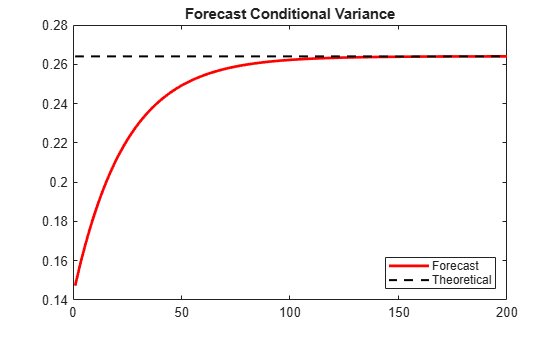Documentation

### This is machine translation

Mouseover text to see original. Click the button below to return to the English version of the page.

Note: This page has been translated by MathWorks. Click here to see
To view all translated materials including this page, select Country from the country navigator on the bottom of this page.

## Forecast a Conditional Variance Model

This example shows how to forecast a conditional variance model using `forecast`.

### Load the data and specify the model.

Load the Deutschmark/British pound foreign exchange rate data included with the toolbox, and convert to returns. For numerical stability, convert returns to percentage returns.

```load Data_MarkPound r = price2ret(Data); pR = 100*r; T = length(r);```

Specify and fit a GARCH(1,1) model.

```Mdl = garch(1,1); EstMdl = estimate(Mdl,pR);```
``` GARCH(1,1) Conditional Variance Model (Gaussian Distribution): Value StandardError TStatistic PValue ________ _____________ __________ __________ Constant 0.010868 0.0012972 8.3779 5.3897e-17 GARCH{1} 0.80452 0.016038 50.162 0 ARCH{1} 0.15433 0.013852 11.141 7.9449e-29 ```

### Generate MMSE forecasts.

Use the fitted model to generate MMSE forecasts over a 200-period horizon. Use the observed return series as presample data. By default, `forecast` infers the corresponding presample conditional variances. Compare the asymptote of the variance forecast to the theoretical unconditional variance of the GARCH(1,1) model.

```v = forecast(EstMdl,200,pR); sig2 = EstMdl.Constant/(1-EstMdl.GARCH{1}-EstMdl.ARCH{1}); figure plot(v,'r','LineWidth',2) hold on plot(ones(200,1)*sig2,'k--','LineWidth',1.5) xlim([0,200]) title('Forecast Conditional Variance') legend('Forecast','Theoretical','Location','SouthEast') hold off```The MMSE forecasts converge to the theoretical unconditional variance after about 160 steps.

Download ebook